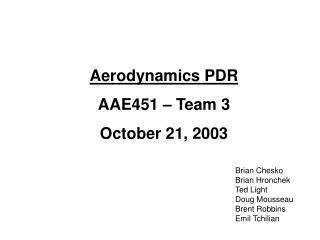DownloadDownload PresentationAerodynamics PDR AAE451 – Team 3 October 21, 2003

# Aerodynamics PDR AAE451 – Team 3 October 21, 2003

Download Presentation## Aerodynamics PDR AAE451 – Team 3 October 21, 2003

- - - - - - - - - - - - - - - - - - - - - - - - - - - E N D - - - - - - - - - - - - - - - - - - - - - - - - - - -
##### Presentation Transcript

1. Aerodynamics PDR AAE451 – Team 3 October 21, 2003 Brian Chesko Brian Hronchek Ted Light Doug Mousseau Brent Robbins Emil Tchilian

2. Aerodynamics PDR Design Process • Span-wise distribution for cl found using Lifting-Line Theory • Airfoil Selection • Drag Integration

3. Lifting-Line Theory • Wing modeled as distribution of horseshoe vortices • Fourier Series for circulation along span Inputs: a = dcl/da = 5.66 (airfoil specific) Constant Chord = 2.8 AR = 5 a = 6.85 deg (to match CL) (actually a - aL=0)

4. Prandtl’s Lifting-Line • Solve Prandtl’s wing equation • Substitute • System of N equations with N unknowns(Solve N  N matix) • Take N different spanwise locations on the wing where the equation is to be satisfied: 1, 2, .. N; (but not at the tips, so: 0 < 1 < )

5. Lifting-Line For Rectangular Wing • Consider: rectangular wing: c = constant; span = b; b/c = A = 5 without twist: = constant; L=0 = 0 • Evaluate the wing equation at the N control points at i : • The wing is symmetrical  A2, A4,… are zero • take only A1, A3,… as unknowns • take only control points on half of the wing: 0 < i  /2 • Example for N=30: • take A1, A3, A5 as unknowns • take control points (equidistant in ):  = /(2N) stepping • take lift-slope of the airfoil a0 = 5.66, and wing aspect ratio A = 5

6. Lifting-Line Calculation • Sample Output N = 3 • CL calculation from lifting line theory CL = πAR*A1*αCL = W/S*q = .4873 from constraint solve for a = 6.8 deg in order to match CL • CDicalculation • clcalculation

7. Lifting-Line Theory Outputs: CDi = 0.0131 Cdi distribution CL = 0.4873 Cl distribution Section Lift Coefficient Varies from ~ 0 – 0.6

8. Airfoil Selection Airfoils Selection Criteria: • Low drag over range of specified cl values • Easy construction • Round Leading Edge • Relatively Flat Bottom • Easy to construct on tabletop • Constructible Trailing Edge

9. Airfoil Selection Region of Interest Clark Y Clark Y Airfoil is Best

10. Clark Y Airfoil • Geometry • Drag Polar • cl vs a cl vs. a cd vs. cl dcl/da = 5.66

11. Total Lift and Drag Coefficient Estimation • Lift: • CL – Found at cruise, can be obtained at any a • cl - Found using lifting line theory • Drag: • CD = CDi + CDp • CDifound using lifting line theory, can be obtained at any a • From Drag Polar of airfoil (cl vs. cd), cdp can be obtained and integrated to obtain CDp for the entire wing

12. Parasitic Drag Calculation • Used Polynomial Function to Fit Airfoil’s Drag Polar

13. Parasitic Drag Calculation • Plugged wing cl distribution into polynomial function to get corresponding parasitic cd distribution along span

14. Parasitic Drag Calculation • Integrated Parasitic Drag Distribution Along Span to get 3-D Wing Parasitic Drag • CDp = .0059

15. Total Wing Drag Coefficient • CD = CDi + CDp • CD = .0131 + .0059 = .0190

16. Wing Characteristics Wing Sweep = 0º Taper Ratio = 1 Dihedral Angle = 5º AR = 5 S = 40 ft2 Tail Airfoil = NACA 0012 (empirically based from Roskam Part II, p. 154) (subject of future trade study)

17. Wing Characteristics

18. Coming Attractions… • CLmax • Control Surface Sizing • Tail Sizing

19. Questions?